#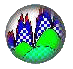Interactive Real Analysis - part of MathCS.org

Next | Previous | Glossary | Map | Discussion

## 4.1. Series and Convergence

So far we have learned about sequences of numbers. Now we will investigate what may happen when we add all terms of a sequence together to form what will be called an infinite series.

The old Greeks already wondered about this, and actually did not have the tools to quite understand it This is illustrated by the old tale of Achilles and the Tortoise.

 Example 4.1.1: Zeno's Paradox (Achilles and the Tortoise) Achilles, a fast runner, was asked to race against a tortoise. Achilles can run 10 meters per second, the tortoise only 5 meter per second. The track is 100 meters long. Achilles, being a fair sportsman, gives the tortoise 10 meter advantage. Who will win ?
• Both start running, with the tortoise being 10 meters ahead.
• After one second, Achilles has reached the spot where the tortoise started. The tortoise, in turn, has run 5 meters.
• Achilles runs again and reaches the spot the tortoise has just been. The tortoise, in turn, has run 2.5 meters.
• Achilles runs again to the spot where the tortoise has just been. The tortoise, in turn, has run another 1.25 meters ahead.
This continues for a while, but whenever Achilles manages to reach the spot where the tortoise has just been a split-second ago, the tortoise has again covered a little bit of distance, and is still ahead of Achilles. Hence, as hard as he tries, Achilles only manages to cut the remaining distance in half each time, implying, of course, that Achilles can actually never reach the tortoise. So, the tortoise wins the race, which does not make Achilles very happy at all.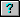Obviously, this is not true, but where is the mistake ?

Now let's return to mathematics. Before we can deal with any new objects, we need to define them:

 Definition 4.1.2: Series, Partial Sums, and Convergence Let { a n } be an infinite sequence. The formal expression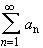is called an (infinite) series. For N = 1, 2, 3, ... the expression lim Sn =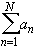is called the N-th partial sum of the series. If lim Sn exists and is finite, the series is said to converge. If lim Sn does not exist or is infinite, the series is said to diverge.
Note that while a series is the result of an infinite addition - which we do not yet know how to handle - each partial sum is the sum of finitely many terms only. Hence, the partial sums form a sequence, and we already know how to deal with sequences.
 Examples 4.1.3: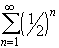= 1/2 + 1/4 + 1/8 + 1/16 + ... is an infinite series. The 3rd, 4th, and 5th partial sums, for example, are, respectively: 0.875, 0.9375, and 0.96875.Does this series converge or diverge ?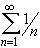= 1 + 1/2 + 1/3 + 1/4 + 1/5 + ... is another infinite series, called harmonic series. The 3rd, 4th, and 5th partial sums are, respectively: 1.833, 2.0833, and 2.2833.Does this series converge or diverge ?
Actually, if a series contains positive and negative terms, many of them may cancel out when being added together. Hence, there are different modes of convergence: one mode that applies to series with positive terms, and another mode that applies to series whose terms may be negative and positive.
 Definition 4.1.4: Absolute and Conditional Convergence A seriesconverges absolutely if the sum of the absolute values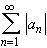converges. A series converges conditionally, if it converges, but not absolutely.
 Examples 4.1.5:Does the series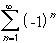converge absolutely, conditionally, or not at all ?Does the series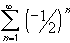converge absolutely, conditionally, or not at all ?Does the series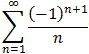converge absolutely, conditionally, or not at all (this series is called alternating harmonic series) ?Show that if a seriesconverges absolutely, it converges in the ordinary sense. The converse is not true.
Conditionally convergent sequences are rather difficult to work with. Several operations that one would expect to be true do not hold for such series. The perhaps most striking example is the associative law. Since a + b = b + a for any two real numbers a and b, positive or negative, one would expect also that changing the order of summation in a series should have little effect on the outcome. However:
 Theorem 4.1.6: Absolute Convergence and Rearrangement Letbe an absolutely convergent series. Then any rearrangement of terms in that series results in a new series that is also absolutely convergent to the same limit. Letbe a conditionally convergent series. Then, for any real number c there is a rearrangement of the series such that the new resulting series will converge to c.
It seems that conditionally convergent series contain a few surprises. As a concrete example, we can rearrange the alternating harmonic series so that it converges to, say, 2.
 Examples 4.1.7: Rearranging the Alternating Harmonic SeriesFind a rearrangement of the alternating harmonic seriesthat is within 0.001 of 2, i.e. show a concrete rearrangement of that series that is about to converge to the number 2.Find a rearrangement of the alternating harmonic seriesthat diverges to positive infinity.
Absolutely convergent series, however, behave just as one would expect.
 Theorem 4.1.8: Algebra on Series Letand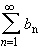be two absolutely convergent series. Then: The sum of the two series is again absolutely convergent. Its limit is the sum of the limit of the two series. The difference of the two series is again absolutely convergent. Its limit is the difference of the limit of the two series. The product of the two series is again absolutely convergent. Its limit is the product of the limit of the two series (Cauchy Product).
We will give one more rather abstract result on series before concluding this chapter as we started it, with a story. The one result that is of more theoretical importance is
 Theorem 4.1.9: Cauchy Criteria for Series The seriesconverges if and only if for every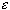> 0 there is a positive integer N such that if m > n > N then |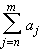| <Our final story is frequently called the "Leaning Tower of Lire". While the introductory story about Achilles and the Tortoise introduces an apparent paradox which we were able to resolve using a convergent (geometric) series, this story uses the properties of a divergent (harmonic) series to shed light on an unbelievable but true situation.

 Example 4.1.10: The Leaning Tower of LireJillian, a diligent but overworked student, fell asleep in the library and got locked in for the night. When she awoke, the room was dimly lit and she was alone. To pass the time (and to annoy the librarian in the morning) she decided to stack books on a table so that they would overhang the edge of the table. Assuming she has an unlimited supply of books, all of equal width 2 and weight 1 (say), what is the biggest overhang she can produce? To make it more interesting, let's say she can use only one book at each level.

But our story-time is over - the next section introduces convenient tests to determine quickly and efficiently whether a series converges or diverges.

Next | Previous | Glossary | Map | Discussion